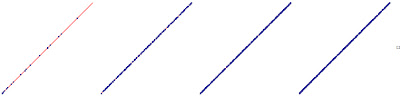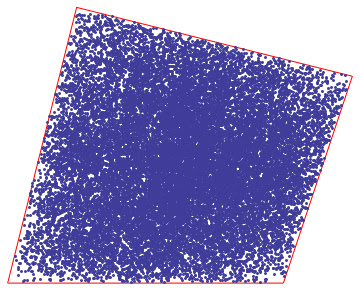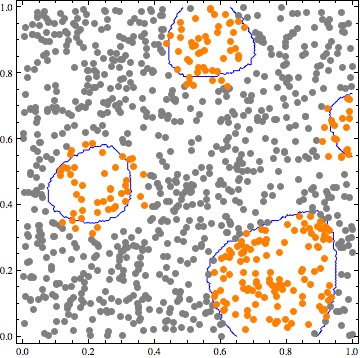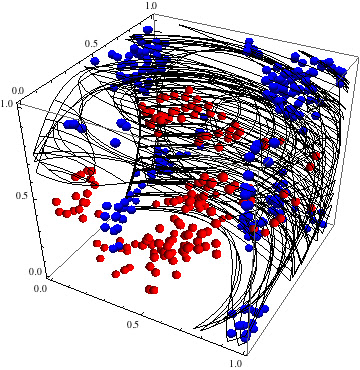Monday, October 24, 2011

Think different part 1: IFS fractals to generate points in a convex polygon

Sometime datasets assume strange shapes, and often to make easier our computations, we simulate the set of points using approximation of well-known statistic distributions, mixture models, and so on, but as we know the world often is more complex than expected, and an analytical density function is tricky to find out.
Let me show, a different approach, to simulate points belonging to really irregular convex polygons.
The method I propose is based on a kind of Markovian Process, better known under the name of Iterated Function System (IFS). The IFS are famous thanks to the “collage theorem” of Prof. M. F. Barnsley that allows amazing image compressions and description of complex phenomena. (I really suggest a deep reading of  the book “Fractal everywhere” that you can buy clicking on the Amazoon panel).
Just to remark the powerful of the IFS fractals, I plotted the famous fern obtained with 4 affine transformations ( I’ll explain below how IFS works).
The sequence of images shows how a fern can be built following the underlying process.IFS to generate the fern. To different transformation has been assigned a different color.
IFS: how it works
To generate an IFS fractal you just need a set of affine contractive transformations and for each transformation an associated probability (…of course the sum of the probabilities associated to the transformations must be equal to one!).
So for a given set of affine contractive transformation {a,b,c,d} and the respective probability set {pr_a, pr_b, pr_c, pr_d} you initialize the process from an arbitrary point of the space “P_t0”, and you find the next point of the process applying to it a transformation chosen among the mentioned set with the associated probability.
That's it!

Generate points in a convex Polygon.
The process to generate a set of points in a convex polygon is based on the above process, but, instead of using a generic affine transformation, we can use only homothetic contractive transformations.
Procedure:
• Find a set of homothetic affine transformations of the polygon having fixed point in the vertexes of the polygon.Homothetic transformations with fixed points on vertexes of the polygon
• Assign equal probability to each transformation
• Trigger the Process starting from a point inside the polygon.
Actually the above procedure works properly also with non concave objects (like straight line):The above procedure applied to find points belonging to a segment
Here you are some examples of set for the above procedures.The set of points obtained for the former polygon. (Changing the contraction factor, you can obtain different coverages).
Others examples:As you can see, some points fall above the polygons: Why? Because I initialized the process from point outside the polygon, but the final effect doesn't change

In the next post we will discuss about the Probability Density Function  (PDF) for the points obtained with this procedure and the relation between the PDF and contractive factor.
Stay Tuned!
cristian

Thursday, October 13, 2011

ADA boost: a way to improve a weak classifier

We have seen that often a classifier is not able to do its job properly.
Why? There are many reasons:
•  Even if many algorithms are in principle general purpose, we know that testing the algorithm on different data set (that is on different problem) the accuracy can change a lot.
• The training hasn’t been done properly: consider for example datasets having high dimensionality, how can you be sure that your classifier hasn’t affected by overfitting?
• The classifier it self cannot solve such problem: e.g a perceptron cannot solve the xor problem (more generally cannot solve non linear problems).

So the idea behind ADA, is to linearly combine a set of weak classifiers to obtain a better classifier!
One of the reasons that makes ADA so powerful is its generality:
It is a meta-algorithm, that is, you can think ADA like a strategy where you can combine different weak classifiers to cooperate together to improve the overall accuracy. So in the linear combination of classifiers you can have perceptrons mixed with neural networks, decision trees and so on.
Every single classifier is specialized to solve just a piece of the problem, so combining them you obtain the overall solution of the problem.
How it works?
ADA is an iterative strategy where on each round, the weights of each incorrectly classified example are increased so that the new classifier focuses more on those examples.
Further details are available everywhere in internet, but I would suggest to focus on the distribution function that it minimizes.
This is a crucial point: the function (at least in the original implementation) is a convex distribution, and the algo tends to fall in local minimum because basically it performs a greedy process.
Again: when you use an algorithm focus on its limits!!
As usual I’m not going to report here the meta-code of algo: there are hundreds of web pages with exactly the same things and I don’t want add entropy to the Universe J
I prefer show the some graphical example done in Mathematica: I believe that an image is thousands times better than a formula to understand the underlying concepts! (…I love the theoretical aspects of the algorithms, but after the theory you must to be practical and able to implement the solution!!).

I ran on the same data set (not linearly separable) many SVM having different setting (of course an SVM well trained can easily solve the problem).
I choose voluntarily a weak kernel (polynomial) with a bad capacity factor values.
Here you are the hyperplanes found by the svm.  As you can see, no one solution found fits properly problem.The set of weak classifier  used to build the boosted classifier

Let me show now what is the output of ADA after few iterations.The output of ADA using the above weak classifiers. Notice how the linear combination produce a segmented classifier.
Below an of example with 3-D datasetsThe set of weak hyperplanes found by the weak svms (mixed)
Here you are the ADA output after just 2 iterations:ADA output after two iterations

and the optimal solution found after 7 iterations:The ADA output after 7 iterations: as you can see the optimal solution has been found.

Contact me if you are interested to the notebook with the Mathematica implementation.
Stay tuned!
cristian